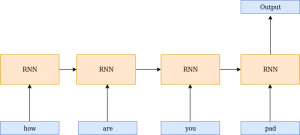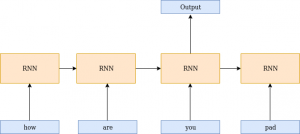# 使用PyTorch建立你的第一个文本分类模型

### 概述

• 学习如何使用PyTorch执行文本分类
• 理解解决文本分类时所涉及的要点

### 介绍1. 为什么使用PyTorch进行文本分类?
– 处理词汇表外单词
– 处理可变长度序列
– 包装器和预训练模型
2. 理解问题
3. 实现文本分类

### 为什么使用PyTorch进行文本分类?

– 动态网络——运行时架构的变化
– 跨gpu的分布式训练#### 2. 处理可变长度序列### 理解问题陈述

Quora希望在他们的平台上追踪不真诚的问题，以便让用户在分享知识的同时感到安全。在这种情况下，一个不真诚的问题被定义为一个旨在发表声明的问题，而不是寻找有用的答案。为了进一步分析这个问题，这里有一些特征可以表明一个特定的问题是不真诚的:

• 语气非中性
• 是贬低还是煽动性的
• 没有现实根据
• 使用性内容(乱伦、兽交、恋童癖)来达到令人震惊的效果，而不是寻求真正的答案

### 实现文本分类

– Torch包用于定义张量和张量上的数学运算
– torchtext是PyTorch中的一个自然语言处理(NLP)库。这个库包含预处理文本的脚本和一些流行的NLP数据集的源。

#导入库
import torch

#处理数据
from torchtext import data


#产生同样的结果
SEED = 2019

#Torch
torch.manual_seed(SEED)

#Cuda 算法
torch.backends.cudnn.deterministic = True


1. field:数据模块中的字段对象用于为数据集中的每一列指定预处理步骤。
2. LabelField: LabelField对象是Field对象的一个特例，它只用于分类任务。它的惟一用途是默认将unk_token和sequential设置为None。

field的参数:
1. Tokenize:指定标记句子的方法，即将句子分词。我正在使用spacy分词器，因为它使用了新的分词算法
2. Lower:将文本转换为小写
batch_first:输入和输出的第一个维度总是批处理大小

fields = [(None, None), ('text',TEXT),('label', LABEL)]


#载入自定义数据集
training_data=data.TabularDataset(path = 'quora.csv',format = 'csv',fields = fields,skip_header = True)

print(vars(training_data.examples))


import random
train_data, valid_data = training_data.split(split_ratio=0.3, random_state = random.seed(SEED))


1. min_freq:忽略词汇表中频率小于指定频率的单词，并将其映射到未知标记。
– unknown标记用于处理词汇表中的单词

#初始化glove embeddings
TEXT.build_vocab(train_data,min_freq=3,vectors = "glove.6B.100d")
LABEL.build_vocab(train_data)

print("Size of TEXT vocabulary:",len(TEXT.vocab))

print("Size of LABEL vocabulary:",len(LABEL.vocab))

print(TEXT.vocab.freqs.most_common(10))

print(TEXT.vocab.stoi)


#检查cuda是否可用
device = torch.device('cuda' if torch.cuda.is_available() else 'cpu')

#设置batch大小
BATCH_SIZE = 64

#载入迭代器
train_iterator, valid_iterator = data.BucketIterator.splits(
(train_data, valid_data),
batch_size = BATCH_SIZE,
sort_key = lambda x: len(x.text),
sort_within_batch=True,
device = device)


#### 模型架构

1. Init:每当创建类的实例时，都会自动调用Init函数。因此，它被称为构造函数。传递给类的参数由构造函数初始化。我们将定义将在模型中使用的所有层
2. Forward: Forward函数定义输入的前向传播。

1. num_embeddings:字典中的单词数量
2. embedding_dim:单词的维度

LSTM: LSTM是RNN的一个变体，能够捕获长期依赖项。遵循你应该熟悉的LSTM的一些重要参数。以下是这一层的参数:
1. input_size:输入的维度
2. hidden_size:隐藏节点的数量
3. num_layers:要堆叠的层数
4. batch_first:如果为真，则输入和输出张量以(batch, seq, feature)的形式提供。
5. dropout:如果非零，则在除最后一层外的每一LSTM层的输出上引入一个dropout层，dropout概率等于dropout。默认值:0
6. bidirection:如果为真，则引入双向LSTM

1. in_features:输入的特征数量
2. out_features:隐藏层的节点数量

import torch.nn as nn

class classifier(nn.Module):

#定义所有层
def __init__(self, vocab_size, embedding_dim, hidden_dim, output_dim, n_layers,
bidirectional, dropout):

super().__init__()

#embedding 层
self.embedding = nn.Embedding(vocab_size, embedding_dim)

#lstm 层
self.lstm = nn.LSTM(embedding_dim,
hidden_dim,
num_layers=n_layers,
bidirectional=bidirectional,
dropout=dropout,
batch_first=True)

#全连接层
self.fc = nn.Linear(hidden_dim * 2, output_dim)

#激活函数
self.act = nn.Sigmoid()

def forward(self, text, text_lengths):

#text = [batch size,sent_length]
embedded = self.embedding(text)
#embedded = [batch size, sent_len, emb dim]

packed_output, (hidden, cell) = self.lstm(packed_embedded)
#hidden = [batch size, num layers * num directions,hid dim]
#cell = [batch size, num layers * num directions,hid dim]

#连接最后的正向和反向隐状态
hidden = torch.cat((hidden[-2,:,:], hidden[-1,:,:]), dim = 1)

#hidden = [batch size, hid dim * num directions]
dense_outputs=self.fc(hidden)

#激活
outputs=self.act(dense_outputs)

return outputs


#定义超参数
size_of_vocab = len(TEXT.vocab)
embedding_dim = 100
num_hidden_nodes = 32
num_output_nodes = 1
num_layers = 2
bidirection = True
dropout = 0.2

#实例化模型
model = classifier(size_of_vocab, embedding_dim, num_hidden_nodes,num_output_nodes, num_layers,
bidirectional = True, dropout = dropout)


#模型体系
print(model)

def count_parameters(model):
return sum(p.numel() for p in model.parameters() if p.requires_grad)

print(f'The model has {count_parameters(model):,} trainable parameters')

#初始化预训练embedding
pretrained_embeddings = TEXT.vocab.vectors
model.embedding.weight.data.copy_(pretrained_embeddings)

print(pretrained_embeddings.shape)


import torch.optim as optim

#定义优化器和损失
criterion = nn.BCELoss()

#定义度量
def binary_accuracy(preds, y):
#四舍五入到最接近的整数
rounded_preds = torch.round(preds)

correct = (rounded_preds == y).float()
acc = correct.sum() / len(correct)
return acc

#如果cuda可用
model = model.to(device)
criterion = criterion.to(device)


1. 训练阶段:model.train()将模型设置在训练阶段，并激活dropout层。
2. 推理阶段:model.eval()将模型设置在评估阶段，并停用dropout层。

def train(model, iterator, optimizer, criterion):

#初始化
epoch_loss = 0
epoch_acc = 0

#设置为训练模式
model.train()

for batch in iterator:

#在每一个batch后设置0梯度

text, text_lengths = batch.text

#转换成一维张量
predictions = model(text, text_lengths).squeeze()

#计算损失
loss = criterion(predictions, batch.label)

#计算二分类精度
acc = binary_accuracy(predictions, batch.label)

#反向传播损耗并计算梯度
loss.backward()

#更新权重
optimizer.step()

#损失和精度
epoch_loss += loss.item()
epoch_acc += acc.item()

return epoch_loss / len(iterator), epoch_acc / len(iterator)


def evaluate(model, iterator, criterion):

#初始化
epoch_loss = 0
epoch_acc = 0

#停用dropout层
model.eval()

for batch in iterator:

text, text_lengths = batch.text

#转换为一维张量
predictions = model(text, text_lengths).squeeze()

#计算损失和准确性
loss = criterion(predictions, batch.label)
acc = binary_accuracy(predictions, batch.label)

#跟踪损失和准确性
epoch_loss += loss.item()
epoch_acc += acc.item()

return epoch_loss / len(iterator), epoch_acc / len(iterator)


N_EPOCHS = 5
best_valid_loss = float('inf')

for epoch in range(N_EPOCHS):

#训练模型
train_loss, train_acc = train(model, train_iterator, optimizer, criterion)

#评估模型
valid_loss, valid_acc = evaluate(model, valid_iterator, criterion)

#保存最佳模型
if valid_loss < best_valid_loss:
best_valid_loss = valid_loss
torch.save(model.state_dict(), 'saved_weights.pt')

print(f'\tTrain Loss: {train_loss:.3f} | Train Acc: {train_acc*100:.2f}%')
print(f'\t Val. Loss: {valid_loss:.3f} |  Val. Acc: {valid_acc*100:.2f}%')


#载入权重
path='/content/saved_weights.pt'
model.eval();

#推理
import spacy

def predict(model, sentence):
tokenized = [tok.text for tok in nlp.tokenizer(sentence)]  #标记句子
indexed = [TEXT.vocab.stoi[t] for t in tokenized]          #转换为整数序列
length = [len(indexed)]
tensor = torch.LongTensor(indexed).to(device)              #转换为tensor
tensor = tensor.unsqueeze(1).T
length_tensor = torch.LongTensor(length)                   #转换为tensor
prediction = model(tensor, length_tensor)                  #预测
return prediction.item()


#作出预测
predict(model, "Are there any sports that you don't like?")

#不真诚的问题
predict(model, "Why Indian girls go crazy about marrying Shri. Rahul Gandhi ji?")


### 结尾